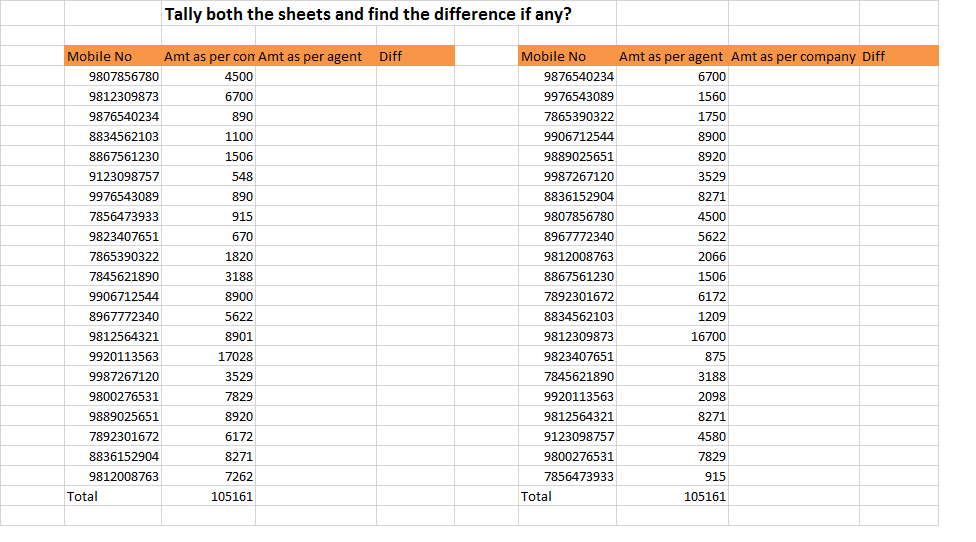Accounts Tax
Excel

Remember we used Vlookup and Hlookup function for

• Bringing data from one sheet to another sheet
• Comparing Data

Same thing can also be done by using combination of INDEX and MATCH function as shown below

#### Formula of INDEX

=INDEX(Select Area ,Write Column No or Row No)

#### Formula of Match

=Match(What to look for,Where to look for,0)

#### Formula of Index and Match

=INDEX(Select Area from where data required,Match(Select Item,Select Area containing Item,0))

Note:-Press F4 while selecting both areas

It is a common formula in place of Vlookup and Hlookup

Note:-

Formula of Index and Formula of Match  are  not very useful to learn individually is

however,combination of both is very important function which we should know and practice

Assignment 1

Re-solve Vlookup Assignment using Index and Match

Assignment 2

Re-solve Hookup Assignment using Index and Match

Assignment 3

Compare two tables using Index and Match#### Advantages of Using Index and Match over Vlookup Hlookup

 VLOOKUP AND HLOOKUP INDEX AND MATCH Vlookup only searches vertically and Hlookup only Horizantallly It can be used for searching both horizantally and vertically Area should be on righr side of item in case of Vlookup and Area should be on downwards item in case of Hlookup No such problem In case,we insert column or row, Vlookup or Hlookup may not work properly No such problem

Get live Maths 1-on-1 Classs - Class 6 to 12